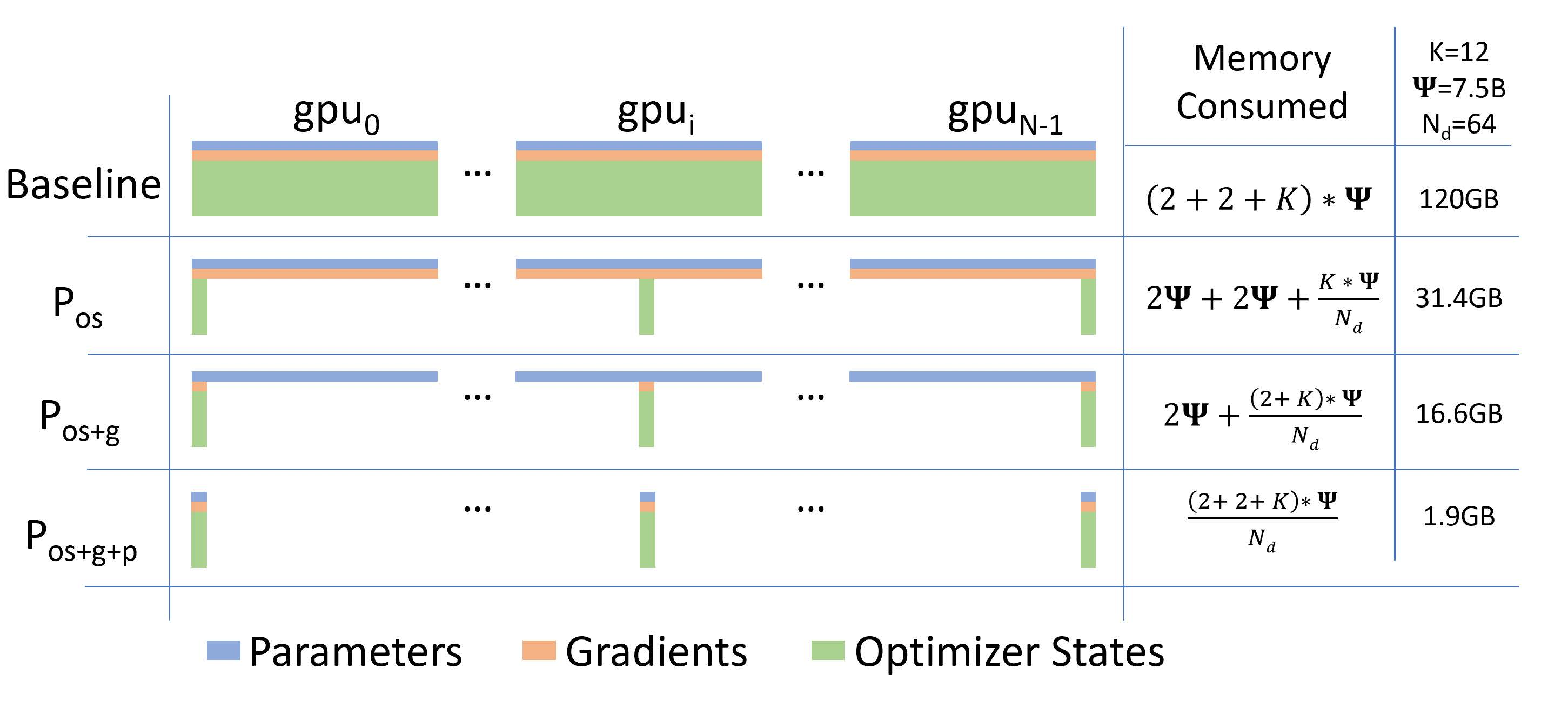# Zero Redundancy Optimizer (ZeRO)¶

## Introduction to ZeRO¶

Zero Redundancy Optimizer (ZeRO) is a method proposed in paper ZeRO: Memory Optimization Towards Training A Trillion Parameter Models, aiming to reduce the memory usage under the data parallelism strategy.

In common data parallelism strategy, each GPU independently maintains a complete set of model parameters, which is efficient in computation and communication, but inefficient in memory. This problem is especially acute when training large models. ZeRO consists of ZeRO-DP and ZeRO-R, which can effectively reduce the consumption of video memory. This means that larger models can be trained with the same amount of memory. It also means that it is possible to use data parallelism for large models that could only be trained with model parallelism strategies in the past.

The memory consumption when training a deep learning model can be divided into two parts:

1. Model States. For large models, most of the memory consumption is occupied by the model state, which mainly includes three parts: Optimizer States, Gradients, and Parameters. The three parts are abbreviated as OPG.

2. Residual States. It includes activation functions, temporary buffers, and unusable memory fragments.

ZeRO-DP can be divided into three stages, eliminating memory redundancy by partitioning the OPG state rather than copying it directly, and each GPU only saves part of the OPG. Specifically, ZeRO-DP has three main optimization stages, corresponding to O, P, and G respectively. The three stages increase step by step:

1. Optimizer states partition（Pos）: This state is 4x less memory consumption and the same amount of traffic as data parallel.
2. Add gradients partition optimizer (Pos+g): At this stage, the memory consumption is reduced by 8 times, and the traffic is the same as the data parallel.
3. Add parameter partition optimizer (Pos+g+p): At this stage, the memory occupied by the model is evenly distributed among each GPU. Memory consumption is linearly inversely proportional to the degree of data parallel, but there will be a slight increase in traffic.

The distribution of the memory consumption of the three stages can be seen in the following figure (from the original ZeRO paper Figure 1):## ZeRo Usage Example¶

First, import OneFlow：

import oneflow as flow
from oneflow import nn


### Definine the Training Process of Data Parallelism¶

We define a training process under a data parallellelism strategy, similar to that described in Conduct data parallel training by setting SBP.

Note

ZeRO can be applied for all the cases where data parallel groups exist. For example, in 2D/3D parallel, ZeRO can be turned on as long as there is a data parallel group.

After the definition, we will use placement, SBP, etc:

P = flow.placement("cuda", ranks=[0, 1])
S0 = flow.sbp.split(0)
DEVICE = "cuda"


For demonstration purposes, we define a simple model and broadcast it to the cluster:

model = nn.Sequential(nn.Linear(256, 128),
nn.ReLU(),
nn.Linear(128, 10))
model = model.to(DEVICE)
model.train()
model = model.to_global(placement=P, sbp=B)

loss_fn = nn.CrossEntropyLoss().to(DEVICE)
optimizer = flow.optim.SGD(model.parameters(), lr=1e-3)


ZeRO is set in the graph compiler of nn.Graph, so the dynamic graph model needs to be converted to nn.Graph:

class CustomGraph(flow.nn.Graph):
def __init__(self):
super().__init__()
self.model = model
self.loss_fn = loss_fn

# TODO: Set ZeRO

def build(self, x, y):
preds = self.model(x)
loss = self.loss_fn(preds, y)
loss.backward()
return preds


Definine the Training Process

graph_model = CustomGraph()

for _ in range(100):
x = flow.randn(128, 256).to(DEVICE)
y = flow.ones(128, 1, dtype=flow.int64).to(DEVICE)
global_x = x.to_global(placement=P, sbp=S0)
global_y = y.to_global(placement=P, sbp=S0)

graph_model(global_x, global_y)


Then start training through launch Module

### Enable ZeRO in nn.Graph¶

ZeRO can be enabled through the interface config.set_zero_redundancy_optimizer_mode .

#### Enable Stage 1 of ZeRO¶

class CustomGraph(flow.nn.Graph):
def __init__(self):
super().__init__()
...
# Enable stage 1 of ZeRO
self.config.enable_zero(True, stage=1)
...


Note

When using the model for continuous training and prediction: After the training is performed once, ZeRO will automatically change the SBP parameter of the model from Broadcast to Split; when performing prediction, Split will be used for automatic inference without configuring ZeRO.

#### Enable Stage 2 of ZeRO¶

class CustomGraph(flow.nn.Graph):
def __init__(self):
super().__init__()
...
# Enable stage 2 of ZeRO
self.config.enable_zero(True, stage=2)
...


Generally speaking, the optimization of stage 2 has large optimization of memory and small speed impact, so it is recommended to use stage 2 optimization. It can be enabled in a simpler way:

class CustomGraph(flow.nn.Graph):
def __init__(self):
super().__init__()
...
# Enable stage 2 of ZeRO
self.config.enable_zero()
...


#### Enable Stage 3 of ZeRO¶

class CustomGraph(flow.nn.Graph):
def __init__(self):
super().__init__()
...
# Enable stage 3 of ZeRO
self.config.enable_zero(True, stage=3)
...


Although enabling the third stage can minimize the memory consumption, it will increase the communication cost which will bring lower speed.